# 【Halcon-1D Measuring】一维测量中measure_pos和measure_pairs算子

首先需要设定测量区域的大小，一般是通过gen_measure_rectangle2 来查找平面区域的边缘距离，通过gen_measure_arc来设定圆弧区域的边缘距离；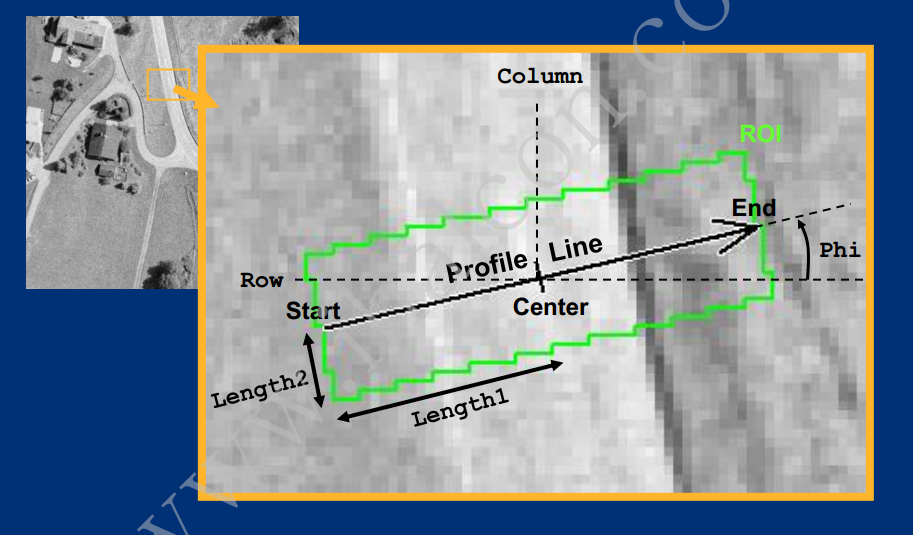Halcon 的F1文档中关于measure_pos有如下说明：

measure_pos(Image : : MeasureHandle, Sigma, Threshold, Transition, Select : RowEdge, ColumnEdge, Amplitude, Distance)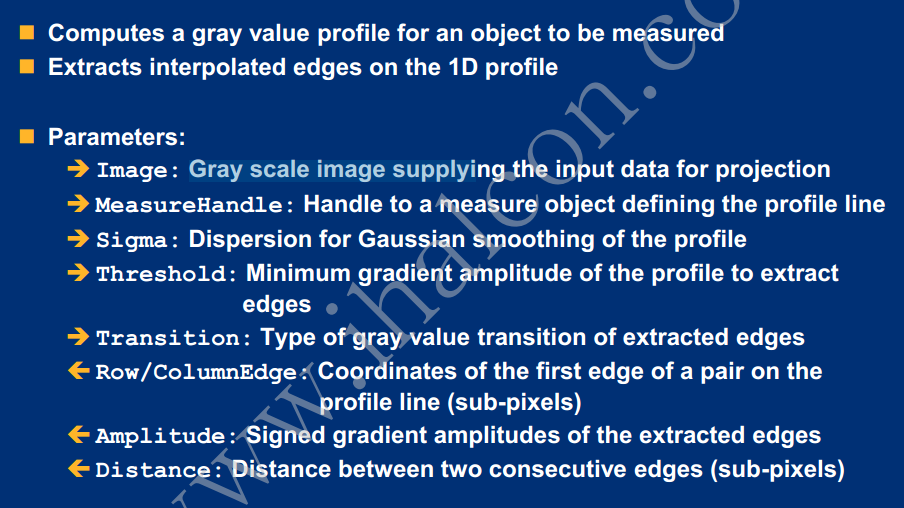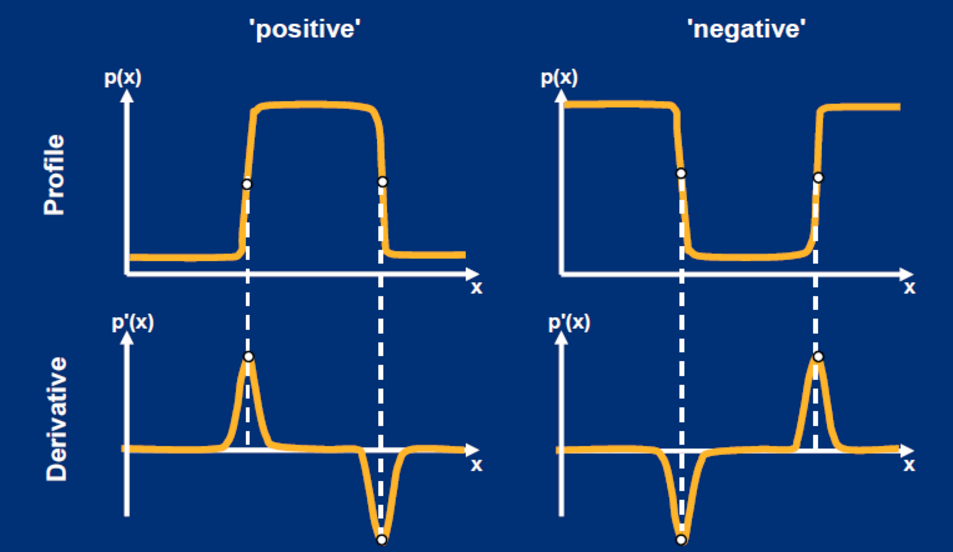以前我理解边缘幅度值边缘处渐变的灰度差值，但现在看来这个理解是不准确的，应该是边缘灰度值变化的趋势，因为由黑到白（小变大）和由白到黑（大变小） 在图形中反映出幅度为负和正；因此可以理解这个幅度值是有正负的；

Threshold 设置的是你选取的幅度阈值下限

Amplitude  获取的是你提取到的边缘处的幅度大小

Transition=‘positive’  代表找寻所有正边缘 ：所谓正边缘即为在查找方向上从黑到白过渡变化的边缘；

Transition=‘negative’  代表找寻所有负边缘 ：所谓负边缘即为在查找方向上从白到黑过渡变化的边缘；

Transition=‘all’  代表找寻所有边缘 ;

measure_pos (Operator)

Name

measure_pos — Extract straight edges perpendicular to a rectangle or annular arc.

Signature

measure_pos(Image : : MeasureHandle, Sigma, Threshold, Transition, Select : RowEdge, ColumnEdge, Amplitude, Distance)

Description

measure_pos extracts straight edges which lie perpendicular to the major axis of a rectangle or annular arc.

For an explanation of the concept of 1D measuring see the introduction of chapter 1D Measuring.

The algorithm of measure_pos works by averaging the gray values in “slices” perpendicular to the major axis of the rectangle or annular arc in order to obtain a one-dimensional edge profile. The sampling is done at subpixel positions in the image Image at integer row and column distances (in the coordinate frame of the rectangle) from the center of the rectangle. Since this involves some calculations which can be used repeatedly in several measurements, the operator gen_measure_rectangle2 or gen_measure_arc is used to perform these calculations only once, thus increasing the speed of measure_pos significantly. Since there is a trade-off between accuracy and speed in the subpixel calculations of the gray values, and thus in the accuracy of the extracted edge positions, different interpolation schemes can be selected in gen_measure_rectangle2. (The interpolation only influences rectangles not aligned with the image axes.) The measure object generated with gen_measure_rectangle2 is passed in MeasureHandle.

After the one-dimensional edge profile has been calculated, subpixel edge locations are computed by convolving the profile with the derivatives of a Gaussian smoothing kernel of standard deviation Sigma. Salient edges can be selected with the parameter Threshold, which constitutes a threshold on the amplitude, i.e., the absolute value of the first derivative of the edge. Note that the amplitude values are scaled by the factor . Additionally, it is possible to select only positive edges, i.e., edges which constitute a dark-to-light transition in the direction of the major axis of the rectangle or the arc (Transition = 'positive'), only negative edges, i.e., light-to-dark transitions (Transition = 'negative'), or both types of edges (Transition = 'all'). Finally, it is possible to select which edge points are returned. If Select is set to 'all', all edge points are returned. If it is set to 'first', only the first of the extracted edge points is returned, while it is set to 'last', only the last one is returned.

The extracted edges are returned as single points which lie on the major axis of the rectangle or arc in (RowEdge,ColumnEdge). The corresponding edge amplitudes are returned in Amplitude. In addition, the distance between consecutive edge points is returned in Distance. Here, Distance[i] corresponds to the distance between Edge[i] and Edge[i+1], i.e., the tuple Distance contains one element less than the tuples RowEdge and ColumnEdge.

Attention

measure_pos only returns meaningful results if the assumptions that the edges are straight and perpendicular to the major axis of the rectangle or arc are fulfilled. Thus, it should not be used to extract edges from curved objects, for example. Furthermore, the user should ensure that the rectangle or arc is as close to perpendicular as possible to the edges in the image. Additionally, Sigma must not become larger than approx. 0.5 * Length1 (for Length1 see gen_measure_rectangle2).

It should be kept in mind that measure_pos ignores the domain of Image for efficiency reasons. If certain regions in the image should be excluded from the measurement a new measure object with appropriately modified parameters should be generated.

Parallelization

Multithreading type: reentrant (runs in parallel with non-exclusive operators).
Processed without parallelization.
Parameters

Image (input_object)  singlechannelimage → object (byte / uint2 / real)
Input image.
MeasureHandle (input_control)  measure_id → (integer)
Measure object handle.
Sigma (input_control)  number → (real)
Sigma of gaussian smoothing.
Default value: 1.0
Suggested values: 0.4, 0.6, 0.8, 1.0, 1.5, 2.0, 3.0, 4.0, 5.0, 7.0, 10.0
Typical range of values: 0.4 ≤ Sigma ≤ 100 (lin)
Minimum increment: 0.01
Recommended increment: 0.1
Restriction: Sigma >= 0.4
Threshold (input_control)  number → (real)
Minimum edge amplitude.
Default value: 30.0
Suggested values: 5.0, 10.0, 20.0, 30.0, 40.0, 50.0, 60.0, 70.0, 90.0, 110.0
Typical range of values: 1 ≤ Threshold ≤ 255 (lin)
Minimum increment: 0.5
Recommended increment: 2
Transition (input_control)  string → (string)
Light/dark or dark/light edge.
Default value: 'all'
List of values: 'all', 'negative', 'positive'
Select (input_control)  string → (string)
Selection of end points.
Default value: 'all'
List of values: 'all', 'first', 'last'
RowEdge (output_control)  point.y-array → (real)
Row coordinate of the center of the edge.
ColumnEdge (output_control)  point.x-array → (real)
Column coordinate of the center of the edge.
Amplitude (output_control)  real-array → (real)
Edge amplitude of the edge (with sign).
Distance (output_control)  real-array → (real)
Distance between consecutive edges.
Result

If the parameter values are correct the operator measure_pos returns the value 2 (H_MSG_TRUE). Otherwise an exception is raised.

Possible Predecessors

gen_measure_rectangle2

Possible Successors

close_measure

Alternatives

edges_sub_pix, fuzzy_measure_pos

measure_pairs, fuzzy_measure_pairs, fuzzy_measure_pairing

Module

1D Metrology

HDevelop例程

measure_pin.hdev        Measure pins of an IC
measure_fill_level.hdev        Inspect the fill level of ampoules
measure_arc.hdev        Measure the width of a metal part along a circular arc
gen_measure_rectangle2.hdev        Measure edges perpendicular to a given line
gen_measure_arc.hdev        Measure edges perpendicular to a given arc
create_drawing_object_rectangle2.hdev        Measure straight edges interactively within a rectangle using a drawing object
3d_coordinates.hdev        Measure slanted object in world coordinates

measure_pairs属于加强版的measure_pos

halcon 的F1文档中关于measure_pairs有如下说明：

measure_pairs(Image : : MeasureHandle, Sigma, Threshold, Transition, Select : RowEdgeFirst, ColumnEdgeFirst, AmplitudeFirst, RowEdgeSecond, ColumnEdgeSecond, AmplitudeSecond, IntraDistance, InterDistance)

1、这个算子找出的都是边缘对（两个边形成一对边缘），边缘给出的是边缘对打间距值

2、边缘查找的方向性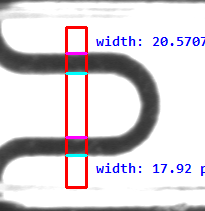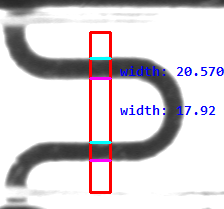3、算子参数Transition 控制着哪种类型的边缘来组成边缘对；

Transition=‘positive’  沿测量矩形中轴线方向（查询方向为MeasureHandle矩形的绘制角度方向），第一个边缘点是 黑-白的点，第二个点是白-黑的点 具有这样特征的边缘将会组成边缘对；

Transition=‘negitive’  沿测量矩形中轴线方向，第一个边缘点是 白-黑的点，第二个点是黑-白的点 具有这样特征的边缘将会组成边缘对；

Transition=‘all’     沿测量矩形中轴线方向，所有具有白黑白 ，或者黑白黑过渡特征的边缘对都会被找出；

边缘对内边缘之间的距离。
5、算子参数 InterDistance
连续边缘对之间的距离。

measure_pairs (Operator)

Name

measure_pairs — Extract straight edge pairs perpendicular to a rectangle or annular arc.

Signature

measure_pairs(Image : : MeasureHandle, Sigma, Threshold, Transition, Select : RowEdgeFirst, ColumnEdgeFirst, AmplitudeFirst, RowEdgeSecond, ColumnEdgeSecond, AmplitudeSecond, IntraDistance, InterDistance)

Description

measure_pairs serves to extract straight edge pairs which lie perpendicular to the major axis of a rectangle or annular arc.

For an explanation of the concept of 1D measuring see the introduction of chapter 1D Measuring.

The extraction algorithm of measure_pairs is identical to measure_pos. In addition the edges are grouped to pairs: If Transition = 'positive', the edge points with a dark-to-light transition in the direction of the major axis of the rectangle are returned in RowEdgeFirst and ColumnEdgeFirst. In this case, the corresponding edges with a light-to-dark transition are returned in RowEdgeSecond and ColumnEdgeSecond. If Transition = 'negative', the behavior is exactly opposite. If Transition = 'all', the first detected edge defines the transition for RowEdgeFirst and ColumnEdgeFirst. I.e., dependent on the positioning of the measure object, edge pairs with a light-dark-light transition or edge pairs with a dark-light-dark transition are returned. This is suited, e.g., to measure objects with different brightness relative to the background.

If more than one consecutive edge with the same transition is found, the first one is used as a pair element. This behavior may cause problems in applications in which the threshold Threshold cannot be selected high enough to suppress consecutive edges of the same transition. For these applications, a second pairing mode exists that only selects the respective strongest edges of a sequence of consecutive rising and falling edges. This mode is selected by appending '_strongest' to any of the above modes for Transition, e.g., 'negative_strongest'. Finally, it is possible to select which edge pairs are returned. If Select is set to 'all', all edge pairs are returned. If it is set to 'first', only the first of the extracted edge pairs is returned, while it is set to 'last', only the last one is returned.

The extracted edges are returned as single points which lie on the major axis of the rectangle. The corresponding edge amplitudes are returned in AmplitudeFirst and AmplitudeSecond. In addition, the distance between each edge pair is returned in IntraDistance and the distance between consecutive edge pairs is returned in InterDistance. Here, IntraDistance[i] corresponds to the distance between EdgeFirst[i] and EdgeSecond[i], while InterDistance[i] corresponds to the distance between EdgeSecond[i] and EdgeFirst[i+1], i.e., the tuple InterDistance contains one element less than the tuples of the edge pairs.

Attention

measure_pairs only returns meaningful results if the assumptions that the edges are straight and perpendicular to the major axis of the rectangle are fulfilled. Thus, it should not be used to extract edges from curved objects, for example. Furthermore, the user should ensure that the rectangle is as close to perpendicular as possible to the edges in the image. Additionally, Sigma must not become larger than approx. 0.5 * Length1 (for Length1 see gen_measure_rectangle2).

It should be kept in mind that measure_pairs ignores the domain of Image for efficiency reasons. If certain regions in the image should be excluded from the measurement a new measure object with appropriately modified parameters should be generated.

Parallelization

Multithreading type: reentrant (runs in parallel with non-exclusive operators).
Processed without parallelization.
Parameters

Image (input_object)  singlechannelimage → object (byte / uint2 / real)
Input image.

MeasureHandle (input_control)  measure_id → (integer)
Measure object handle.

Sigma (input_control)  number → (real)
Sigma of gaussian smoothing.

Default value: 1.0

Suggested values: 0.4, 0.6, 0.8, 1.0, 1.5, 2.0, 3.0, 4.0, 5.0, 7.0, 10.0

Typical range of values: 0.4 ≤ Sigma ≤ 100 (lin)

Minimum increment: 0.01

Recommended increment: 0.1

Restriction: Sigma >= 0.4

Threshold (input_control)  number → (real)
Minimum edge amplitude.

Default value: 30.0

Suggested values: 5.0, 10.0, 20.0, 30.0, 40.0, 50.0, 60.0, 70.0, 90.0, 110.0

Typical range of values: 1 ≤ Threshold ≤ 255 (lin)

Minimum increment: 0.5

Recommended increment: 2

Transition (input_control)  string → (string)
Type of gray value transition that determines how edges are grouped to edge pairs.

Default value: 'all'

List of values: 'all', 'all_strongest', 'negative', 'negative_strongest', 'positive', 'positive_strongest'
Select (input_control)  string → (string)
Selection of edge pairs.

Default value: 'all'

List of values: 'all', 'first', 'last'
RowEdgeFirst (output_control)  point.y-array → (real)
Row coordinate of the center of the first edge.

ColumnEdgeFirst (output_control)  point.x-array → (real)
Column coordinate of the center of the first edge.

AmplitudeFirst (output_control)  real-array → (real)
Edge amplitude of the first edge (with sign).

RowEdgeSecond (output_control)  point.y-array → (real)
Row coordinate of the center of the second edge.

ColumnEdgeSecond (output_control)  point.x-array → (real)
Column coordinate of the center of the second edge.

AmplitudeSecond (output_control)  real-array → (real)
Edge amplitude of the second edge (with sign).

Distance between edges of an edge pair.

InterDistance (output_control)  real-array → (real)
Distance between consecutive edge pairs.

Result

If the parameter values are correct the operator measure_pairs returns the value 2 (H_MSG_TRUE). Otherwise an exception is raised.

Possible Predecessors

gen_measure_rectangle2

Possible Successors

close_measure

Alternatives

edges_sub_pix, fuzzy_measure_pairs, fuzzy_measure_pairing

measure_pos, fuzzy_measure_pos

Module

1D Metrology

HDevelop例程

world_coordinates_line_scan.hdev        Measure distances between the pitch lines of a caliper rule in a line scan image using camera calibration
two_camera_calibration.hdev        Perform high precision mosaicking using a special calibration object
pm_measure_board.hdev        Locate IC on a board and measure pin distances
measure_tft_cells.hdev        Measure the dimensions of the RGB cells of a TFT LCD display
measure_switch.hdev        Determine the width of and the distance between the pins of a switch
measure_ring.hdev        Determine the width of cogs with a circular measure object
measure_pin.hdev        Measure pins of an IC
measure_lcd_cells.hdev        Measure the dimensions of frames that separate the cells of an LCD display
gen_measure_rectangle2.hdev        Measure edges perpendicular to a given line
fuzzy_measure_switch.hdev        Determine the width of and the distance between the pins of a switch with a fuzzy measure object
fuse.hdev        Measure the thickness of a fuse wire
correct_measure_direction.hdev        Automatically correct the orientation of a measure object
camera_calibration_multi_image.hdev        Calibrate the camera and measures positions on a caliper rule
camera_calibration_external.hdev        Measure positions on a caliper rule using camera calibration
calibration_aimdpm_1_2006.hdev        Apply a reflectance calibration before inspecting the print quality of 2D data codes
calibrate_cameras_telecentric_tilt.hdev        Calibrate a setup with a telecentric tilt lens (Scheimpflug)
align_measurements.hdev        Inspect individual razor blades using shape-based matching to align ROIs for the measure tool

---------------------

07-097005
10-11109
08-202019
12-12341
04-242502
08-14925
02-072498
05-201万+
11-151万+
04-276703
05-051177
07-06427
10-13384
04-061921
07-06845
02-251369
08-054489
12-072700
12-123万+

### “相关推荐”对你有帮助么？

•非常没帮助
•没帮助
•一般
•有帮助
•非常有帮助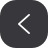被折叠的  条评论 为什么被折叠?到【灌水乐园】发言¥2 ¥4 ¥6 ¥10 ¥20余额支付 (余额：-- )扫码支付获取中扫码支付点击重新获取扫码支付1.余额是钱包充值的虚拟货币，按照1:1的比例进行支付金额的抵扣。
2.余额无法直接购买下载，可以购买VIP、C币套餐、付费专栏及课程。余额充值